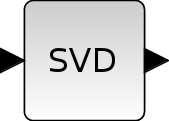Scilab Home page | Wiki | Bug tracker | Forge | Mailing list archives | ATOMS | File exchange
Change language to: English - Français - Português - 日本語 -
Справка Scilab >> Xcos > palettes > Matrix operation palette > MATSING

MATSING

SVD Decomposition

Block ScreenshotDescription

The MATSING block computes the economy sized SVD of the MxN input matrix A by finding U,S and V such that

A=U*S*V'.

When the decomposition type parameter is set to;

• 1 : the output is a vector composed by the singular values.

• 2 : we have three outputs: the second output is a diagonal matrix S composed by the singular values and the other two outputs are the unitary matrices U and V.

The equivalent function of this block in Scilab is svd(A,"e").

Parameters• Datatype(1=real double 2=Complex)

It indicates the type of the output. It support only the two types double (1) and complex (2). If we input another entry in this label xcos will print the message "Datatype is not supported".

Properties : Type 'vec' of size 1.

• decomposition type (1=singular values 2=sing values+matrix U & V)

It indicates the form of the output. When it is set to one, we have a unique vector output (singular values). When it is set to two we have three same sizes matrices(U,S,V).

Properties : Type 'vec' of size 1.

Default properties

• always active: no

• direct-feedthrough: yes

• zero-crossing: no

• mode: no

• regular inputs:

- port 1 : size [-1,-2] / type 1

• regular outputs:

- port 1 : size [-1,1] / type 1

• number/sizes of activation inputs: 0

• number/sizes of activation outputs: 0

• continuous-time state: no

• discrete-time state: no

• object discrete-time state: no

• name of computational function: mat_sing

Interfacing function

• SCI/modules/scicos_blocks/macros/MatrixOp/MATSING.sci

Computational function

• SCI/modules/scicos_blocks/src/c/mat_sing.c
• SCI/modules/scicos_blocks/src/c/mat_svd.c
• SCI/modules/scicos_blocks/src/c/matz_sing.c
• SCI/modules/scicos_blocks/src/c/matz_svd.c

• MATEIG — Matrix Eigenvalues
• MATLU — LU Factorization
• svd — singular value decomposition